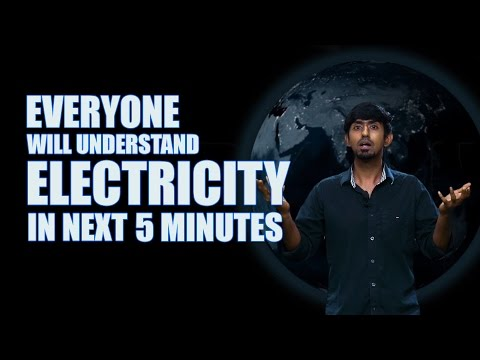# Electromagnetic Field

An electromagnetic field is referred as a physical field of objects that are electrically charged. It is one among the four fundamental forces of nature, other includes strong interaction, weak interaction, and gravitation.

The electromagnetic field affects the change in the behaviour of charged objects surrounding a particular place. It extends indefinitely throughout space and known as electromagnetic interactions.It is a combination of electric field and a magnetic field and often considered as the sources of the electromagnetic field. The electric field is generated by stationary charges, while the magnetic field is produced by moving charges. Here you can find out the difference between electric field and magnetic field.

Lorentz Force Law and Maxwell’s Equation explains how the interaction between currents and charges occurs with the electromagnetic field.

### Electromagnetic Field Structure:

The structure of electromagnetic field can be classified in two distinct methods: Continuous Structure and Discrete Structure.

#### Continuous Structure:

Magnetic and electric fields are produced by an even motion of charged objects. The oscillating charges that produce magnetic and electric fields can be observed in a continuous, smooth and wavelike motion. The energy is observed as being continuous transfer between two electromagnetic field locations.

#### Discrete Structure:

Several experiments has revealed that in a particular circumstance the electromagnetic energy flows in a fixed frequency or a more harsh way in case of a photon. The Planck’s link the photon energy to its frequency. The equation is given below.

$$E = hv$$

Here,

$$\small E$$ = Photon Energy

$$\small h$$ = Planck’s Constant

$$\small v$$ = Frequency of Photon

### Sources of Electromagnetic Fields:

#### Natural Sources:

The electromagnetic field can see everywhere in the surrounding, but they are invisible to human eye. The electric fields are produced by the electric charges that are present in the environment associated with thunderstorms. While the magnetic field of the earth causes the compass needle in North-South Direction. This is used as navigation for fish and birds.